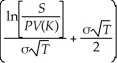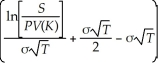# [Solved] Consider the Following Equation: C = S × N

Question 22
Multiple Choice

## Consider the following equation: C = S × N- PV(K)× NIn this equation,the term S represents:

A)the current price of the stock.
B)the stock price at expiration.
C)the annual volatility of the stock.
D)strike price for the option.

10+ million students use Quizplus to study and prepare for their homework, quizzes and exams through 20m+ questions in 300k quizzes.

Explore our library and get Finance Homework Help with various study sets and a huge amount of quizzes and questions

3.5K

Study sets

66.5K

Quizzes

5.9M

Questions

Upload material to get free accessInvite a friend and get free accessSubscribe and get an instant access